# Angle Addition Postulate: Definition & Examples

An error occurred trying to load this video.

Try refreshing the page, or contact customer support.

Coming up next: Proving Angle Relationships

### You're on a roll. Keep up the good work!

Replay
Your next lesson will play in 10 seconds
• 0:01 Angle Addition…
• 1:22 Textbook Definition
• 2:17 Examples
• 4:35 Lesson Summary

Want to watch this again later?

Timeline
Autoplay
Autoplay
Speed

#### Recommended Lessons and Courses for You

Lesson Transcript
Instructor: Miriam Snare

Miriam has taught middle- and high-school math for over 10 years and has a master's degree in Curriculum and Instruction.

In this lesson, you will learn the definition of the Angle Addition Postulate. We will look at some examples so that you understand how this postulate works.

## Angle Addition Postulate Defined

The main idea behind the Angle Addition Postulate is that if you place two angles side by side, then the measure of the resulting angle will be equal to the sum of the two original angle measures. For this postulate to apply, the vertices, which are the corner points of the angle, have to also be placed together. We can illustrate this idea by using the heads of two arrows. We are going to label the arrowheads with some points to make it easier to name the angles.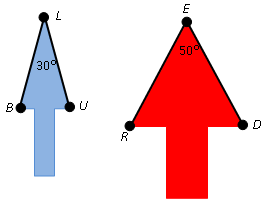In the diagram above, the tip of the blue arrow forms angle BLU which measures 30 degrees. The tip of the red arrow forms angle RED which measures 50 degrees. Now, we are going to add these two angles together by rotating the arrows so that one side of angle BLU is against one side of angle RED and the points at the tips (points L and E) come together. For simplicity, we will call the point at the tips just L.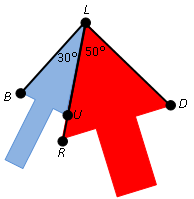Looking at the outside edges of the two arrowheads, there is a new angle that has been created: angle RLU. This angle has a measure of 80 degrees because it was created by joining a 30-degree angle and a 50-degree angle without any space between them and without overlapping them. Below, you can see angle RLU with just the shadows of the two added angles in it.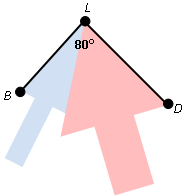## Textbook Definition

Earlier, we looked at how the Angle Addition Postulate physically combines two angles. In a geometry textbook, you often find the Angle Addition Postulate written like this:

If point B lies in the interior of angle AOC, then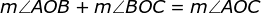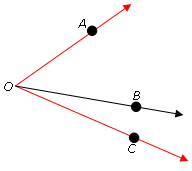Let's start by looking at the diagram to explain the first part of the theorem. Angle AOC is created by the two red rays. In the interior of angle AOC is point B. A ray is drawn from point O through B, which splits angle AOC into two parts (angle AOB and angle BOC). The formula in the theorem tells us that if we add the measures of the two parts (angle AOB and angle BOC) together, we get the measure of the big red angle (angle AOC).

## Examples

Now, let's look at a couple of examples that apply the Angle Addition Postulate.

Example 1: Use the diagram below to find the measure of angle GEM if angle GEO measures 158 degrees and angle MEO is a right angle.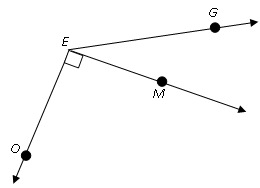So, let's think through the Angle Addition Postulate. What is the name of the big angle in the diagram? What are the names of the two smaller angles that combine to create the big angle?

Angle GEO is the big angle that is made up of angles GEM and MEO. So, we can write the formula from the Angle Addition Postulate for these angles: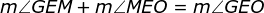Angle MEO measures 90 degrees because it is a right angle. Angle GEO measures 158 degrees as was given in the problem statement. We can substitute in those values into the equation: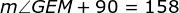Now, we have an equation that we just need to solve for the measure of angle GEM. So, we subtract 90 from both sides. Therefore, angle GEM measures 68 degrees.

Let's try another example.

Example 2: Use the diagram below to find the value of x.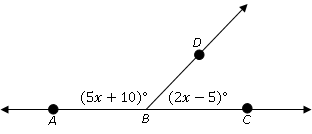To unlock this lesson you must be a Study.com Member.

### Register to view this lesson

Are you a student or a teacher?

### Unlock Your Education

#### See for yourself why 30 million people use Study.com

##### Become a Study.com member and start learning now.
Back
What teachers are saying about Study.com

### Earning College Credit

Did you know… We have over 160 college courses that prepare you to earn credit by exam that is accepted by over 1,500 colleges and universities. You can test out of the first two years of college and save thousands off your degree. Anyone can earn credit-by-exam regardless of age or education level.